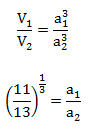# SSC Quantitative Aptitude Questions (Day-71)

Dear Aspirants, you can find the Quantitative Aptitude questions with detailed explanations for the SSC exams. Nowadays the competitive level of the exam has been increasing consistently. Due to the great demand for the government job, the level of the toughness reached greater. Candidates have to enhance the preparation process in order to drive in the right path. It doesn’t need to clear the prescribed cutoff. You must have to score good marks more than the cut off marks to get into the final provisional list. Here we have updating the Quantitative Aptitude questions with detailed explanations on a daily basis. You can practice with us and measure your level of preparation. According to that you can sculpt yourself in a proper way. SSC aspirants kindly make use of it and grab your success in your career.

Start Quiz

1) If interest being compound half yearly then what sum (in Rs) will amount to Rs 38416 in 2 years at the rate of 80% per annum at compound interest?

(a) 14000

(b) 15000

(c) 10000

(d) 12000

Direction (2-5): The table given below shows the number of students studying in five colleges in the given five years.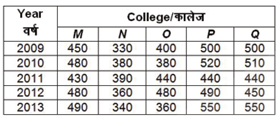2) If in year 2011, in college N, 80% of the total students appeared in a exam, out of which 50% students passed, then how many students passed the exam?

(a) 136

(b) 152

(c) 156

(d) 162

3) What is the ratio of the total number of students of college N in all years to the total number of students of all the colleges styding in the year 2011?

(a)100 : 107

(b) 90 : 107

(c) 90 : 119

(d) 90 : 127

4) What is the average of the total number of students studying in college M in the given years?

(a) 412

(b) 420

(c) 400

(d) 466

5) In which of the given years the average number of students studying is maximum?

(a) 2011

(b) 2009

(c) 2010

(d) 2013

6) The perimeter of a rhombus in 20 cm and one of the diagonal is 8 cm. what is the area (in cm2) of the rhombus?

(a) 12

(b) 24

(c) 48

(d) 96

7) Three circles of radius 9 cm are kept touching each other. The string is tightly tied around the three circles. What is the length (in cm) of the string?

(a) 48 + 18π

(b) 48 + 24π

(c) 54 + 18π

(d) 54 + 24π

8) The difference between circumference and the radius of a circle is 111 cm. What is the area (in cm2) of the circle?

(a) 469

(b) 1386

(c) 912

(d) 1086

9) If the diameter of a sphere is 14 cm, then what is the curved surface area (in cm2) of the sphere?

(a) 616

(b) 1232

(c) 2464

(d) 576

10) If the ratio of volume of two cubes is 11: 13, then what is the ratio of the sides of the two cubes?

(a) 11 : 13

(b) 121 : 169

(c) (11)1/2 : (13)1/2

(d) (11)1/3 : (13)1/3

let the original sum = 100

For 1 yr = 40 + 40 + (40 × 40) / 100
= 96%

For 2 yrs = 96 +  96 + (96 × 96)/100

= 192 + 92.16

= 284.16%

Total sum after two years = 100 + 284.16 =384.16%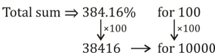= ½ × 80/100 × 390

= 0.4 × 390

= 156

Ntotal=1800

No. of students in year 2011 ⇒ 2140

Ratio = 1800 : 2140

90:  107

MTotal=2330/5 = 466

Total students in year

2009  ⇒ 2180

2010  ⇒2270

2011  ⇒2140

2012  ⇒2260

2013  ⇒2290

Hence average no. of students was maximum in 2013.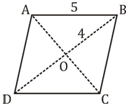∵ diagonal of rhombus intersect at right angle hence

AO = 3 cm ⇒ AC = 6 cm

Area of rhombus = ½ ×6 × 8 = 24 cm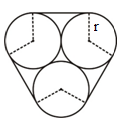Curved string = 2πr

= 18π cm

Straight string = 3 × (28) = 54 cm

Total length of string = 54 + 18π cm

(2π – 1) r = 111

(44/7–1) r = 111

37/7 r = 111

r = 21 cm

Area of circle = 22/7 × 21 × 21

=1386 cm2

d = 14 cm

r = 7 cm

C.S.A. of a sphere = 4πr²

= 4 × 22/7 × 7 × 7

= 616 cm2